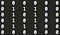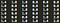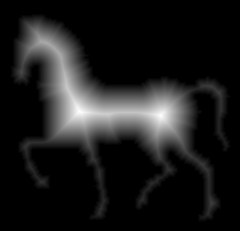# High-level explanation

I'm going to briefly and informally describe one of my favorite image operators, the Euclidean Distance Transform (EDT, for short). When I refer to "image" in this article, I'm referring to a 2D image. Also, the distance referred in this article refers to the Euclidean distance between two points. If you are looking for a high-level introduction on image operators using graphs, this may be right article for you.

The simplest Distance Transform , receives as input a binary image as Figure 1, (the pixels are either 0 or 1), and outputs a distance map (Figure 2). This distance map has the same dimensions of the input image and each pixel contains for example, the Euclidean distance, to the closest obstacle pixel (e.g. border pixel).Figure 1. Binary Image as input.Figure 2. Distance map. Each pixel contains the distance to the closets border pixel (Number 1 on the above image).

One can be interested on computing the distance map only on the inner side of an object. Figure 3 show this as an example. All distances were normalized to be between the [0, 255] interval.Figure 3. EDT of a natural image. The original image needs to be binarized. The border image of this binary image is the input for the EDT algorithm. The result was normalized between the interval [0, 255]. 

On natural greyscale images, you will need to binarize the image (e.g using a threshold method), generate the border image. The border image is a binary image with value 1 on the border and 0 at everything else. This border image will be the input for the EDT algorithm.

# Definitions

An image can be interpreted as a graph. Every pixel is a vertex and the edges are defined by an adjacency relation.

The most succinct explanation of adjacent pixels: set of pixels that share a property. In our context, this property will be the Euclidean distance between them. For a given pixel p, any other pixel that it is within a distance square root of 2, is adjacent to p. This is also know as 8-neighbor adjacency, meaning that the 8 neighbors surrounding p are adjacent to it.

Therefore, given an image with n pixels and an 8-neighbor adjacency relation, it can be interpreted as graph of n vertices and at most 8n edges.

## Paths

A path is another important definition. Path is a sequence of pixels (t1, t2, …, tn), where (ti, t(i+1)) are adjacent. A path is said trivial, if it contains only itself.

A path cost function f, is a function that assigns each path, a cost. Usually this cost depends on local image properties (e.g, color or gradient, … ).

A path is said optimum if its cost is the smallest, possible, considering all other possible paths irrespective of its starting point.

## Image Foresting Transform

A dynamic algorithm called Image Foresting Transform  (IFT) receives as input an image, an adjacency relation and a cost function. It produces as output an optimum-path forest. Since we are looking for the distance to the closest border pixel to a given pixel, the IFT algorithm perform this minimization in O(n). Linear on the number of pixels.

A very briefly description of the algorithm before the code: A wave propagates from special pixels called seeds (which are trivial paths) to all the others pixels on the image. For each neighbor of the current pixel, a value, resultant from the cost function, is offered. If the adjacent pixel "receives a better offer", meaning that it will minimize its current value, the neighbor pixel update its value and sets the new closest border pixel.

Just by modeling the cost function and choosing the appropriate seed set, this same algorithm can be used to develop several image operators , such as the Watershed transform; iterative relative fuzzy connectedness; region clustering for superpixel generation; among others.

# EDT Method

Our cost function has two scenarios. If the path is trivial or it is a path extension, meaning that a new pixel may become a segment on a path.

• If the path is trivial, the distance from a pixel to itself, has cost zero.
• If it is a path extension, the cost is the Euclidean distance from the pixel being evaluated to the closets border pixel of its neighbor.

From now on, try to visualize the EDT operator code following these instructions, it is really easy! You will feel like coding while you read.

A variable image that holds the input binary image. Recalling, an image with only zeros and ones. The ones are border pixels, the zeros are regular black pixels.

A variable distance that holds the current cost assigned at each pixel. Obviously this array has the same size of the image array declared on the paragraph above. Since we want to minimize the cost function, each pixel starts with the highest possible value, let's say: 4294967296. (2^32)

A variable roots that holds the position of the closest border pixel for each pixel. With the same dimensions as the image and distance variable. In this declaration section, all positions are empty, let's say 4294967296 again.

Just as traversing a regular graph iteratively, we will need a queue. There you go, now you have a queue and a 8-neighbor adjacency relation.

Now we need to set the appropriates values for the seeds pixels. We want our wave propagation to start from the border pixels onward. So we make a `for` loop on the image array. If the ith pixel is a border pixel, then its distance is zero (obeying the cost function), its root is set to itself and it is inserted on the queue.

Propagate! While there is still a pixel on the queue, remove it. Traverse through its neighbors pixels.

Is the Euclidean distance between my root and my neighbor smaller then the current cost of my neighbor? (cost function disguised as a question)

If so, the new cost of my neighbor is this distance. My root is the new root of my neighbor. Since the propagation needs to continue my neighbor is inserted on the queue.

That's it! When the queue is empty, all pixels were processed and the distance variable holds the closest distance to the border pixel. We could also create a variable predecessor and store the predecessor for each pixel until it reaches a border pixel with predecessor equal nil. This would provide us the actual "optimum-path", but this is not relevant on this example of the IFT Algorithm.

The complete python code, with comments, can be found here: EDT Method

# Applications

## Error Metric

A distance map can be used as a error metric of segmentation algorithms. The EDT is computed on the border image of the ground truth. For each border pixel on the label image resultant from the delineation algorithm that you are evaluating, sum the distance on the distance map computed from the ground truth and divide by the number of border pixels on the label image. This will give an average distance from the surface of your segmentation to the correct segmentation. A perfect segmentation will produce error zero, since all border pixels are perfectly on the same position. If this is made both ways, it is called: average symmetric absolute surface distance .

## Erosion and Dilation

One can compute the erosion or the dilation of a binary image using the EDT. For a perfect square structuring element, you can propagate the distance transform until the current distance reaches a certain radius r. This radius can be inward or outward, depending if it is erosion or dilation. This would yield to a faster algorithm compared to convolution.

Definitely there are several others applications that I can not recall now. Explore!

# Wrapping up

I hope this article left you curious about using graphs on image processing. If you are interested on formal proof of correctness or efficiency, I recommend reading the official Image Foresting Transform paper . Please mind that this is a high-level explanation, several details were omitted for simplicity.

# References

Written by

Written by

## Nikolas Moya

#### MSc Computer Scientist. Developer at Work and Co.## More From Medium

Welcome to a place where words matter. On Medium, smart voices and original ideas take center stage - with no ads in sight.
Follow all the topics you care about, and we’ll deliver the best stories for you to your homepage and inbox.
Get unlimited access to the best stories on Medium — and support writers while you’re at it. Just \$5/month.